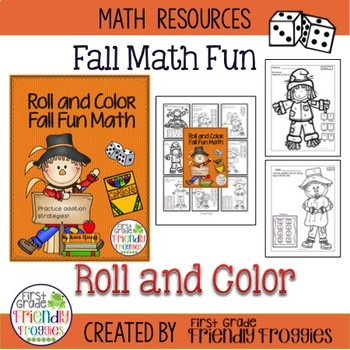K - 1st
Subjects
Standards
Resource Type
Formats Included
• PDF
Pages
13 pages

### Description

Use DICE during math time and turn worksheets into GAMESHEETS! This product includes nine Roll, Add and Color Scarecrow themed worksheets! Your students roll the dice, perform mental math practice with strategies and color the answer! Worksheets use 1 or 2 dice! Make learning fun for your students.

Includes:
directions
9worksheets

Use these for independent practice or in math tubs!

You may also enjoy:
Thanksgiving Fill in the 100s Chart

Total Pages
13 pages
N/A
Teaching Duration
N/A
Report this Resource to TpT
Reported resources will be reviewed by our team. Report this resource to let us know if this resource violates TpT’s content guidelines.

### Standards

to see state-specific standards (only available in the US).
Determine the unknown whole number in an addition or subtraction equation relating three whole numbers. For example, determine the unknown number that makes the equation true in each of the equations 8 + ? = 11, 5 = ▯ - 3, 6 + 6 = ▯.
Add and subtract within 20, demonstrating fluency for addition and subtraction within 10. Use strategies such as counting on; making ten (e.g., 8 + 6 = 8 + 2 + 4 = 10 + 4 = 14); decomposing a number leading to a ten (e.g., 13 - 4 = 13 - 3 - 1 = 10 - 1 = 9); using the relationship between addition and subtraction (e.g., knowing that 8 + 4 = 12, one knows 12 - 8 = 4); and creating equivalent but easier or known sums (e.g., adding 6 + 7 by creating the known equivalent 6 + 6 + 1 = 12 + 1 = 13).
When counting objects, say the number names in the standard order, pairing each object with one and only one number name and each number name with one and only one object.